# WavePage 1

#### WATCH ALL SLIDES

Slide 1Slide 2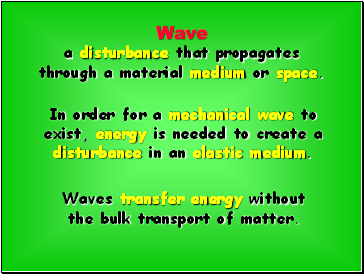## Wave

a disturbance that propagates

through a material medium or space.

Waves transfer energy without

the bulk transport of matter.

In order for a mechanical wave to exist, energy is needed to create a disturbance in an elastic medium.

Slide 3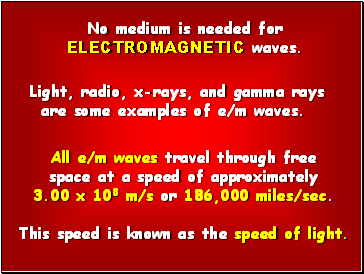All e/m waves travel through free

space at a speed of approximately

3.00 x 108 m/s or 186,000 miles/sec.

This speed is known as the speed of light.

Light, radio, x-rays, and gamma rays

are some examples of e/m waves.

No medium is needed for

ELECTROMAGNETIC waves.

Slide 4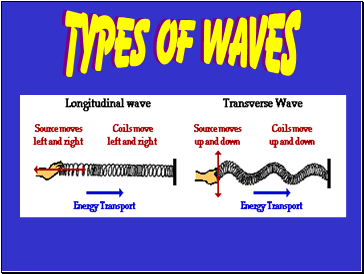Slide 5The displacement of the particles

of the medium is perpendicular to

the direction of wave propagation.

TRANSVERSE

LONGITUDINAL

The displacement of the particles

of the medium is parallel to the

direction of wave propagation.

Slide 6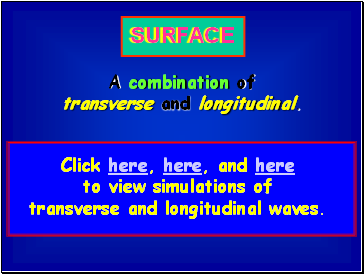to view simulations of

transverse and longitudinal waves.

SURFACE

A combination of

transverse and longitudinal.

Slide 7Amplitude

the maximum displacement

of a particle of the medium from

the rest or equilibrium position

denoted by A and measured in units of length

Slide 8Phase

related to the position and motion

of the particles of the medium

Slide 9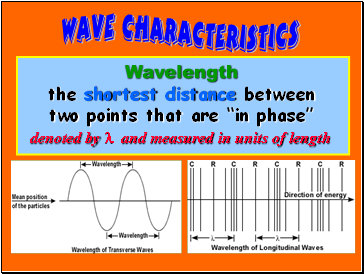Wavelength

the shortest distance between

two points that are “in phase”

denoted by l and measured in units of length

Slide 10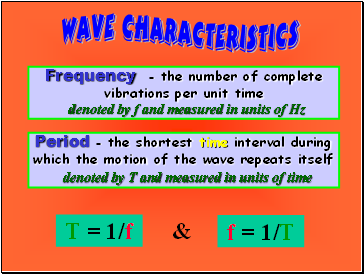Frequency - the number of complete vibrations per unit time

denoted by f and measured in units of Hz

Period - the shortest time interval during

which the motion of the wave repeats itself

denoted by T and measured in units of time

T = 1/f

&

f = 1/T

Slide 11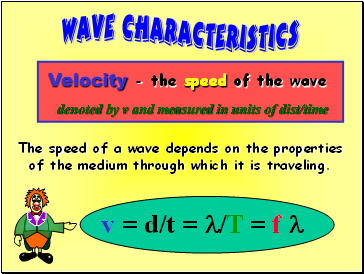Velocity - the speed of the wave

denoted by v and measured in units of dist/time

v = d/t = l/T = f l

The speed of a wave depends on the properties

of the medium through which it is traveling.

Slide 12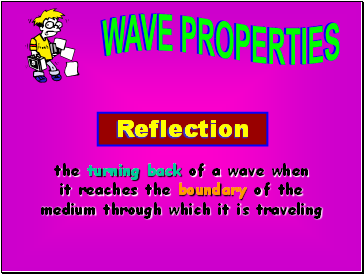Go to page:
1  2  3